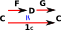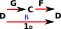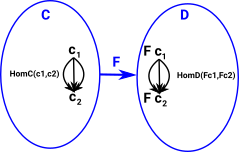# Maths - Category Theory - Weak Equivilance

Weak equivilance provides a simpler way to determine equivilance.

 On the equivilance page we discussed how to determine equivilance by finding two functors. F and G, which give the identity when composed in both directions.Weak equivilance gives us a way to do this using only a functor in one direction F. However this only tells us that an equivilance exists, not the functors which give the equivilance.Given this functor F : C -> D there is an equivalence if and only if the following are all true:

• F is full, i.e. for any two objects c1 and c2 of C, the map HomC(c1,c2) -> HomD(Fc1,Fc2) induced by F is surjective;
• F is faithful, i.e. for any two objects c1 and c2 of C, the map HomC(c1,c2) -> HomD(Fc1,Fc2) induced by F is injective; and
• F is essentially surjective (dense), i.e. each object d in D is isomorphic to an object of the form Fc, for c in C.

## Weak Factorisation System

#### Example in Set

In a set category where morphisms are functions. Each function can be factored into injective and surjective (epi and monic) functions.

Or we can look at this as fibre and co-fibre. More about fibres on page here.The square commutes, the function from A to D factors so there must be a unique arrow (shown dotted) to connect them.

#### Example in Simplical Set

Here all functions are (weakly) order preserving. These can be factored into degenerate arrows (which increase dimension) and arrows which remove a dimension.

More about simplical sets on page here.

## Further Information

Weak equivalence was first used in algebraic topology, in particular, in model category theory as described on the page here.